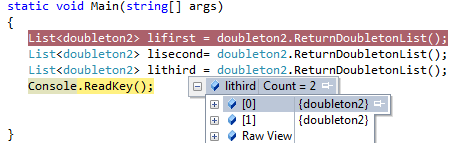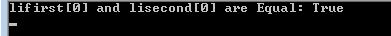I would like to share a way by which we can create a class which could create maximum two objects.

This could be extension of Singleton pattern.

I would like to introduce following way to create doubleton class.

##### Code Sample
`sealed class doubleton2    {        private static readonly object doubletonLock = new object();        private static int index = 0;        private static List<doubleton2> list = new List<doubleton2>();          private doubleton2() { }         public static List<doubleton2> ReturnDoubletonList()         {                     lock (doubletonLock)             {                 if (index < 2)                 {                     list.Add(new doubleton2());                     index++;                 }              }             return list;          }       }       class Program    {        static void Main(string[] args)        {           List<doubleton2> lifirst = doubleton2.ReturnDoubletonList();           List<doubleton2> lisecond= doubleton2.ReturnDoubletonList();           List<doubleton2> lithird = doubleton2.ReturnDoubletonList();           Console.ReadKey();         }    } `

##### Understanding the code

a.       We created List object of  doubleton2 class

b.      On each call of ReturnDoubletonList() method we are just incrementing the

value of this static field (index integer)

c.       ReturnDoubletonList () method is static and it returns  List object of

Doubleton class

d.      We have index<2 inside ReturnDoubletonList () method to make sure we can

have maximum two objects of doubleton class.

##### After executing first line on main function
`List<doubleton2> lifirst = doubleton2.ReturnDoubletonList();We have a list of one object.`1. After executing second line on main function

`List<doubleton2> liSecond = doubleton2.ReturnDoubletonList();We have a list of two objects.`1. After executing third line on main function

`List<doubleton2> lithird = doubleton2.ReturnDoubletonList();Again we have a list of two objects because we have index<2 check in ReturndoubetonList function.`##### Facts with the code

Here in above code following things could be note down.

1. Object lifirst is always equal to lisecond.
2. If I added following line to main function

Console.WriteLine("lifirst and lisecond are Equal: " +

lifirst.Equals(lisecond));

##### Then following would be output.##### Conclusion

From above way we can learn how to create doubleton class. Similarly we can N-

ton class as well.

We can modify this class as per requirement.

Modified On Sep-07-2019 12:14:43 AM
1.Well explained... Waiting for more knowledgeable articles from your side